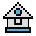Home

# CVI, CVS, CVD Functions

#### Purpose:

To convert string values to numeric values.

#### Syntax:

```CVI(2-byte string)
CVS(4-byte string)
CVD(8-byte string)```

Numeric values read in from a random-access disk file must be converted from strings back into numbers if they are to be arithmetically manipulated.

CVI converts a 2-byte string to an integer. MKI\$ is its complement.

CVS converts a 4-byte string to a single-precision number. MKS\$ is its complement.

CVD converts an 8-byte string to a double-precision number. MKD\$ is its complement.

(See MKI\$, MKS\$, and MKD\$).

#### Examples:

```.
.
.
70 FIELD #1, 4 AS N\$, 12 AS B\$...
80 GET #1
90 Y=CVS(N\$)
.
.
.```

Line 80 reads a field from file #1 (the field read is defined in line 70), and converts the first four bytes (N\$) into a single-precision number assigned to the variable Y.

Since a single-precision number can contain as many as seven ASCII characters (seven bytes), writing a file using MKS\$ conversion, and reading with the CVS conversion, as many as three bytes per number recorded are saved on the storage medium. Even more may be saved if double-precision numbers are required. MKD\$ and CVD conversions would be used in this case.